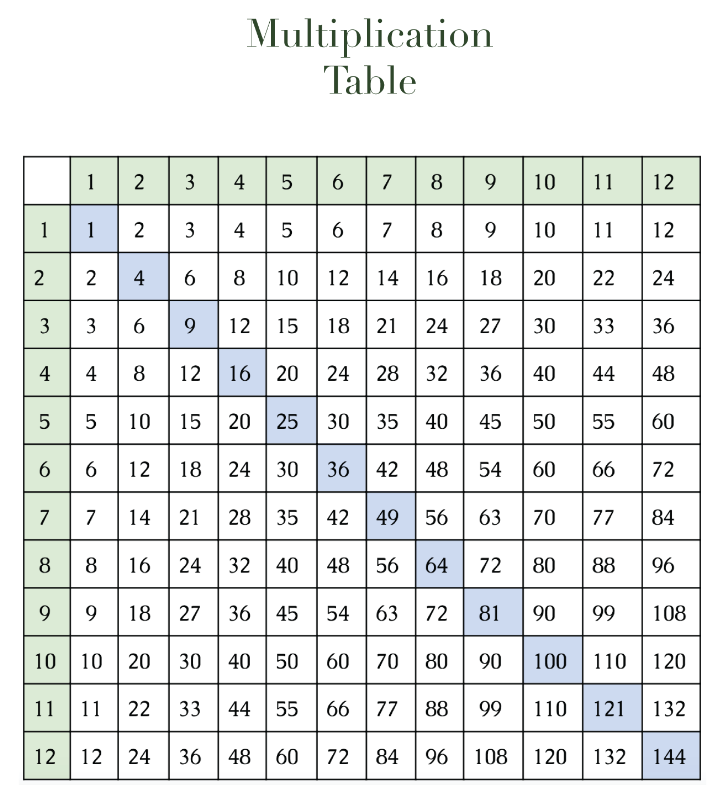We get it. Teaching children (or yourself) isn’t the most exciting thing to do. We understand that students have different learning styles so we’ve created several more designs and chart styles in different colors and formats that hopefully will resonate with you and make learning your times tables a lot easier (and fun!)

## Printable times tables

Our times tables and charts allow you to practice multiplying the basic multiplication equations in a fun and colorful way.

This next times table allows you to match numbers together and see how they multiply together. If you follow one column or row across, it gives how one number is multiplied up to 10. The blue diagonal gives squared numbers (a number multiplied by itself).In this next times table, we give you all the ways a number is multiplied up to 12. Going past 10 is a bit more difficult for kids to memorize, but most kids will have to learn up to 12, so it’s good to get them started on recognizing these patterns.### Print out times tables and charts

Teachers and students alike can utilize our pre-filled times tables worksheet to get their students to memorize and recall the products of basic multiplication of numbers up to 10 (or 12). You can download the above two charts in PDF below.
1. Multiplication table with diagonal answers: PDF
2. Printable times table 12 x 12: PDF